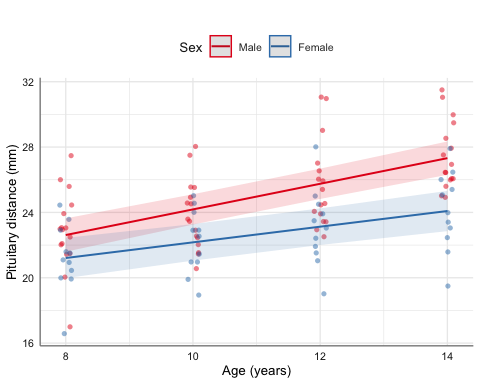# 1 Introduction

The aim of this vignette is to illustrate the use/functionality of the glm_coef function. glm_coef can be used to display model coefficients with confidence intervals and p-values. The advantages and limitations of glm_coef are:

1. Recognises the main models used in epidemiology/public health.
2. Automatically back-transforms estimates and confidence intervals, when the model requires it.
3. Can use robust standard errors for the calculation of confidence intervals.
• Standard errors are used by default.
• The use of standard errors is restricted by the following classes of objects (models): gee, glm and survreg.
4. Can display nice labels for the names of the parameters.
5. Returns a data frame that can be modified and/or exported as tables for publications (with further editing).

We start by loading relevant packages and setting the theme for the plots (as suggested in the Template of this package):

rm(list = ls())
library(car)
library(broom)
library(tidyverse)
library(ggfortify)
library(mosaic)
library(huxtable)
library(jtools)
library(latex2exp)
library(pubh)
library(sjlabelled)
library(sjPlot)
library(sjmisc)

theme_set(sjPlot::theme_sjplot2(base_size = 10))
theme_update(legend.position = "top")
# options('huxtable.knit_print_df' = FALSE)
options('huxtable.knit_print_df_theme' = theme_article)
options('huxtable.autoformat_number_format' = list(numeric = "%5.2f"))
knitr::opts_chunk$set(collapse = TRUE, comment = NA) # 2 Multiple Linear Regression For continuous outcomes there is no need of exponentiating the results unless the outcome was fitted in the log-scale. In our first example we want to estimate the effect of smoking and race on the birth weight of babies. We can generate factors and assign labels in the same pipe stream: data(birthwt, package = "MASS") birthwt <- birthwt %>% mutate( smoke = factor(smoke, labels = c("Non-smoker", "Smoker")), race = factor(race, labels = c("White", "African American", "Other")) ) %>% var_labels( bwt = 'Birth weight (g)', smoke = 'Smoking status', race = 'Race' ) Is good to start with some basic descriptive statistics, so we can compare the birth weight between groups. birthwt %>% group_by(race, smoke) %>% summarise( n = n(), Mean = mean(bwt, na.rm = TRUE), SD = sd(bwt, na.rm = TRUE), Median = median(bwt, na.rm = TRUE), CV = rel_dis(bwt) ) summarise() regrouping output by 'race' (override with .groups argument) # A tibble: 6 x 7 # Groups: race  race smoke n Mean SD Median CV <fct> <fct> <int> <dbl> <dbl> <dbl> <dbl> 1 White Non-smoker 44 3429. 710. 3593 0.207 2 White Smoker 52 2827. 626. 2776. 0.222 3 African American Non-smoker 16 2854. 621. 2920 0.218 4 African American Smoker 10 2504 637. 2381 0.254 5 Other Non-smoker 55 2816. 709. 2807 0.252 6 Other Smoker 12 2757. 810. 3146. 0.294 From the previous table, the group with the lower birth weight was from babies born from African Americans who were smokers. The highest birth weight was from babies born from White non-smokers. Another way to compare the means between the groups is with gen_bst_df which estimates means with corresponding bootstrapped CIs. birthwt %>% gen_bst_df(bwt ~ race|smoke) Birth weight (g)LowerCIUpperCIRaceSmoking status 3428.753195.353634.14WhiteNon-smoker 2826.852654.223004.10WhiteSmoker 2854.502570.713152.76African AmericanNon-smoker 2504.002094.422870.57African AmericanSmoker 2815.782634.943003.48OtherNon-smoker 2757.172275.973137.51OtherSmoker Another approach to tabular analysis is graphical analysis. For this comparison, box-plots would be the way to go, but in health sciences it is more common to see bar charts with error bars. birthwt %>% bar_error(bwt ~ race, fill = ~ smoke) %>% axis_labs() %>% gf_labs(fill = "Smoking status:")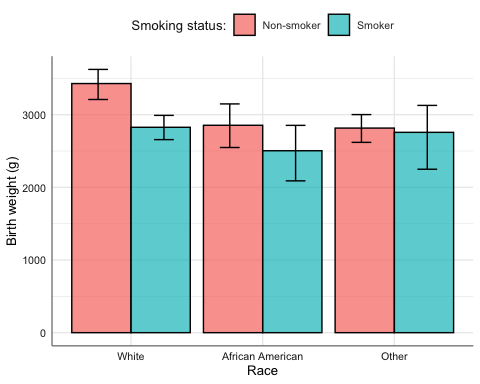We fit a linear model. model_norm <- lm(bwt ~ smoke + race, data = birthwt) Note: Model diagnostics are not be discussed in this vignette. Traditional output from the model: model_norm %>% Anova() %>% tidy() termsumsqdfstatisticp.value smoke7322574.73 1.0015.46 0.00 race8712354.03 2.00 9.20 0.00 Residuals87631355.83185.00 model_norm %>% tidy() termestimatestd.errorstatisticp.value (Intercept)3334.9591.7836.34 0.00 smokeSmoker-428.73109.04-3.93 0.00 raceAfrican American-450.36153.12-2.94 0.00 raceOther-452.88116.48-3.89 0.00 Table of coefficients: model_norm %>% glm_coef(labels = model_labels(model_norm)) ParameterCoefficientPr(>|t|) Constant3334.95 (3153.89, 3516.01)< 0.001 Smoking status: Smoker-428.73 (-643.86, -213.6)< 0.001 Race: African American-450.36 (-752.45, -148.27)0.004 Race: Other-452.88 (-682.67, -223.08)< 0.001 Note: Compare results using robust standard errors. model_norm %>% glm_coef(se_rob = TRUE, labels = model_labels(model_norm)) ParameterCoefficientPr(>|t|) Constant3334.95 (3144.36, 3525.53)< 0.001 Smoking status: Smoker-428.73 (-652.88, -204.58)< 0.001 Race: African American-450.36 (-734.09, -166.63)0.002 Race: Other-452.88 (-701.4, -204.35)< 0.001 The function glance from the broom package allow us to have a quick look at statistics related with the model. model_norm %>% glance() r.squaredadj.r.squaredsigmastatisticp.valuedflogLikAICBICdeviancedf.residual 0.12 0.11688.25 8.68 0.004-1501.113012.223028.4387631355.83185 To construct the effect plot, we can use plot_model from the sjPlot package. The advantage of plot_model is that recognises labelled data and uses that information for annotating the plots. model_norm %>% plot_model("pred", terms = ~race|smoke, dot.size = 1.5, title = "")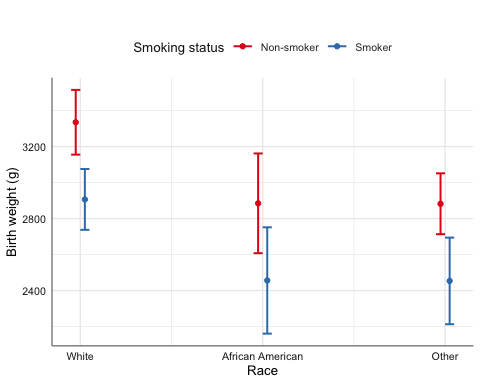When the explanatory variables are categorical, another option is emmip from the emmeans package. We can include CIs in emmip but as estimates are connected, the resulting plots look more messy, so I recommend emmip to look at the trace. emmip(model_norm, smoke ~ race) %>% gf_labs(y = get_label(birthwt$bwt), x = "", col = "Smoking status")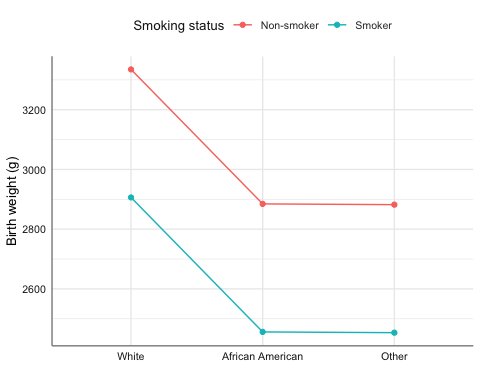# 3 Logistic Regression

For logistic regression we are interested in the odds ratios. We will look at the effect of amount of fibre intake on the development of coronary heart disease.

data(diet, package = "Epi")
diet <- diet %>%
mutate(
chd = factor(chd, labels = c("No CHD", "CHD"))
) %>%
var_labels(
chd = "Coronary Heart Disease",
fibre = "Fibre intake (10 g/day)"
)

diet %>% estat(~ fibre|chd)
Coronary Heart DiseaseNMin.Max.MeanMedianSDCV
Fibre intake (10 g/day)No CHD288.00 0.60 5.35 1.75 1.69 0.58 0.33
CHD45.00 0.76 2.43 1.49 1.51 0.40 0.27

It is standard to plot the dependent variable in the $$y$$-axis, so in this case, we can use horizontal box-plots.

diet %>%
gf_boxploth(chd ~ fibre, fill = "indianred3", alpha = 0.7) %>%
axis_labs()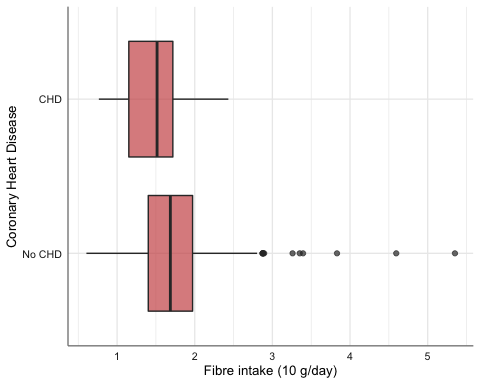We fit a linear logistic model:

model_binom <- glm(chd ~ fibre, data = diet, family = binomial)

model_binom %>%
glm_coef(labels = model_labels(model_binom))
ParameterOdds ratioPr(>|z|)
Constant0.95 (0.29, 3.11) 0.94
Fibre intake (10 g/day)0.33 (0.15, 0.69) 0.00

Effect plot:

model_binom %>%
plot_model("pred", terms = "fibre [all]", title = "")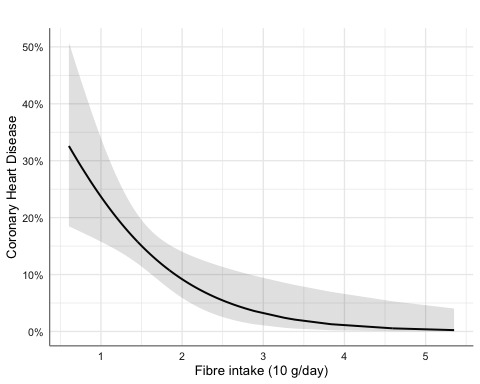## 3.1 Matched Case-Control Studies: Conditional Logistic Regression

We will look at a matched case-control study on the effect of oestrogen use and history of gall bladder disease on the development of endometrial cancer.

data(bdendo, package = "Epi")
bdendo <- bdendo %>%
mutate(
cancer = factor(d, labels = c('Control', 'Case')),
gall = factor(gall, labels = c("No GBD", "GBD")),
est = factor(est, labels = c("No oestrogen", "Oestrogen"))
) %>%
var_labels(
cancer = 'Endometrial cancer',
est = 'Oestrogen'
)

bdendo %>%
mutate(
cancer = relevel(cancer, ref = "Case"),
est = relevel(est, ref = "Oestrogen"),
gall = relevel(gall, ref = "GBD")
) %>%
copy_labels(bdendo) %>%
cross_tab(cancer ~ est + gall) %>%
theme_article()
Endometrial cancer
CaseControlTotal
(N=63)(N=252)(N=315)
Oestrogen
- Oestrogen 56 (88.9%)127 (50.4%)183 (58.1%)
- No oestrogen 7 (11.1%)125 (49.6%)132 (41.9%)
- GBD 17 (27.0%)24 ( 9.5%)41 (13.0%)
- No GBD 46 (73.0%)228 (90.5%)274 (87.0%)

We fit the conditional logistic model:

library(survival)
model_clogit <- clogit(cancer == 'Case'  ~ est * gall + strata(set), data = bdendo)

model_clogit %>%
glm_coef(labels = c("Oestrogen/No oestrogen", "GBD/No GBD",
"Oestrogen:GBD Interaction")) 
ParameterOdds ratioPr(>|z|)
Oestrogen/No oestrogen14.88 (4.49, 49.36)< 0.001
GBD/No GBD18.07 (3.2, 102.01)0.001
Oestrogen:GBD Interaction0.13 (0.02, 0.9)0.039

Creating data frame needed to construct the effect plot:

require(ggeffects)
bdendo_pred <- ggemmeans(model_clogit, terms = c('gall', 'est'))

Effect plot:

bdendo_pred %>%
gf_pointrange(predicted + conf.low + conf.high ~ x|group, col = ~ x) %>%
levels(quine$Sex) <- c("Female", "Male") quine <- quine %>% var_labels( Days = "Number of absent days", Eth = "Ethnicity", Age = "Age group" ) Descriptive statistics: quine %>% group_by(Eth, Sex, Age) %>% summarise( n = n(), Mean = mean(Days, na.rm = TRUE), SD = sd(Days, na.rm = TRUE), Median = median(Days, na.rm = TRUE), CV = rel_dis(Days) ) summarise() regrouping output by 'Eth', 'Sex' (override with .groups argument) # A tibble: 16 x 8 # Groups: Eth, Sex  Eth Sex Age n Mean SD Median CV <fct> <fct> <fct> <int> <dbl> <dbl> <dbl> <dbl> 1 Aboriginal Female F0 5 17.6 17.4 11 0.987 2 Aboriginal Female F1 15 18.9 16.3 13 0.865 3 Aboriginal Female F2 9 32.6 27.3 20 0.839 4 Aboriginal Female F3 9 14.6 14.9 10 1.02 5 Aboriginal Male F0 8 11.5 7.23 12 0.629 6 Aboriginal Male F1 5 9.6 4.51 7 0.469 7 Aboriginal Male F2 11 30.9 17.8 32 0.576 8 Aboriginal Male F3 7 27.1 10.4 28 0.382 9 White Female F0 5 19.8 9.68 20 0.489 10 White Female F1 17 7.76 6.48 6 0.834 11 White Female F2 10 5.7 4.97 4 0.872 12 White Female F3 10 13.5 11.5 12 0.851 13 White Male F0 9 13.6 20.9 7 1.54 14 White Male F1 9 5.56 5.39 5 0.970 15 White Male F2 10 15.2 12.9 12 0.848 16 White Male F3 7 27.3 22.9 27 0.840 We start by fitting a standard Poisson linear regression model: model_pois <- glm(Days ~ Eth + Sex + Age, family = poisson, data = quine) model_pois %>% glm_coef(labels = model_labels(model_pois), se_rob = TRUE) ParameterRate ratioPr(>|z|) Constant17.66 (11.08, 28.16)< 0.001 Ethnicity: White0.59 (0.43, 0.81)0.001 Sex: Male1.11 (0.81, 1.52)0.51 Age group: F10.8 (0.48, 1.32)0.38 Age group: F21.42 (0.87, 2.31)0.16 Age group: F31.35 (0.81, 2.24)0.255 model_pois %>% glance() null.deviancedf.nulllogLikAICBICdeviancedf.residual 2073.53145-1165.492342.982360.881742.50140 ## 4.1 Negative-binomial The assumption is that the mean is equal than the variance. If that is the case, deviance should be close to the degrees of freedom of the residuals (look at the above output from glance). In other words, the following calculation should be close to 1: deviance(model_pois) / df.residual(model_pois)  12.44646 Thus, we have over-dispersion. One option is to use a negative binomial distribution. library(MASS) Attaching package: 'MASS' The following objects are masked _by_ '.GlobalEnv': birthwt, housing, quine The following object is masked from 'package:dplyr': select model_negbin <- glm.nb(Days ~ Eth + Sex + Age, data = quine) model_negbin %>% glm_coef(labels = model_labels(model_negbin), se_rob = TRUE)  ParameterRate ratioPr(>|z|) Constant20.24 (12.72, 32.21)< 0.001 Ethnicity: White0.57 (0.41, 0.78)< 0.001 Sex: Male1.07 (0.78, 1.45)0.688 Age group: F10.69 (0.42, 1.16)0.16 Age group: F21.2 (0.72, 2)0.492 Age group: F31.29 (0.74, 2.23)0.369 model_negbin %>% glance() null.deviancedf.nulllogLikAICBICdeviancedf.residual 192.24145-547.831109.651130.54167.86140 Notice that age group is a factor with more than two levels and is significant: model_negbin %>% Anova() LR ChisqDfPr(>Chisq) 12.66 1.00 0.00 0.15 1.00 0.70 9.48 3.00 0.02 Thus, we want to report confidence intervals and $$p$$-values adjusted for multiple comparisons. Effect plot: model_negbin %>% plot_model(type = "pred", terms = c("Age", "Eth"), dot.size = 1.5, title = "")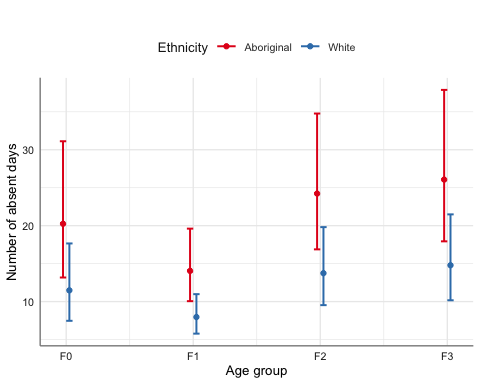emmip(model_negbin, Eth ~ Age|Sex) %>% gf_labs(y = "Number of absent days", x = "Age group", col = "Ethnicity")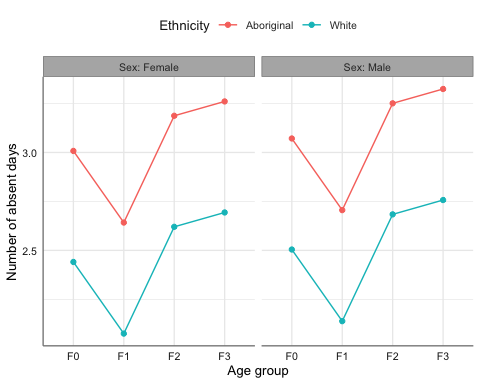## 4.2 Adjusting CIs and p-values for multiple comparisons We adjust for multiple comparisons: multiple(model_negbin, ~ Age|Eth)$df 
contrastEthratioSEz.ratiop.valuelower.CLupper.CL
F1 / F0Aboriginal 0.69 0.16-1.57 0.40 0.38 1.26
F2 / F0Aboriginal 1.20 0.28 0.77 0.86 0.66 2.17
F2 / F1Aboriginal 1.73 0.35 2.65 0.04 1.02 2.92
F3 / F0Aboriginal 1.29 0.31 1.04 0.72 0.69 2.40
F3 / F1Aboriginal 1.86 0.40 2.89 0.02 1.07 3.21
F3 / F2Aboriginal 1.08 0.23 0.34 0.99 0.62 1.88
F1 / F0White 0.69 0.16-1.57 0.40 0.38 1.26
F2 / F0White 1.20 0.28 0.77 0.86 0.66 2.17
F2 / F1White 1.73 0.35 2.65 0.04 1.02 2.92
F3 / F0White 1.29 0.31 1.04 0.72 0.69 2.40
F3 / F1White 1.86 0.40 2.89 0.02 1.07 3.21
F3 / F2White 1.08 0.23 0.34 0.99 0.62 1.88

We can see the comparison graphically with:

multiple(model_negbin, ~ Age|Eth)$fig_ci %>% gf_labs(x = "IRR")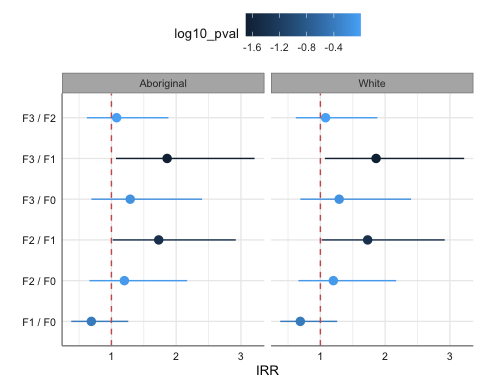# 5 Survival Analysis We will use an example on the effect of thiotepa versus placebo on the development of bladder cancer. data(bladder) bladder <- bladder %>% mutate(times = stop, rx = factor(rx, labels=c("Placebo", "Thiotepa")) ) %>% var_labels(times = "Survival time", rx = "Treatment") ## 5.1 Parametric method model_surv <- survreg(Surv(times, event) ~ rx, data = bladder) Using robust standard errors: model_surv %>% glm_coef(labels = c("Treatment: Thiotepa/Placebo", "Scale"), se_rob = TRUE) ParameterSurvival time ratioPr(>|z|) Treatment: Thiotepa/Placebo1.64 (0.89, 3.04)0.116 Scale1 (0.85, 1.18)0.992 In this example the scale parameter is not statistically different from one, meaning hazard is constant and thus, we can use the exponential distribution: model_exp <- survreg(Surv(times, event) ~ rx, data = bladder, dist = "exponential") model_exp %>% glm_coef(labels = c("Treatment: Thiotepa/Placebo"), se_rob = TRUE) ParameterSurvival time ratioPr(>|z|) Treatment: Thiotepa/Placebo1.64 (0.85, 3.16)0.139 Interpretation: Patients receiving Thiotepa live on average 64% more than those in the Placebo group. Using naive standard errors: model_exp %>% glm_coef(labels = c("Treatment: Thiotepa/Placebo")) ParameterSurvival time ratioPr(>|z|) Treatment: Thiotepa/Placebo1.64 (1.11, 2.41)0.012 model_exp %>% plot_model(type = "pred", terms = ~ rx, dot.size = 1.5, title = "") %>% gf_labs(y = "Survival time", x = "Treatment", title = "")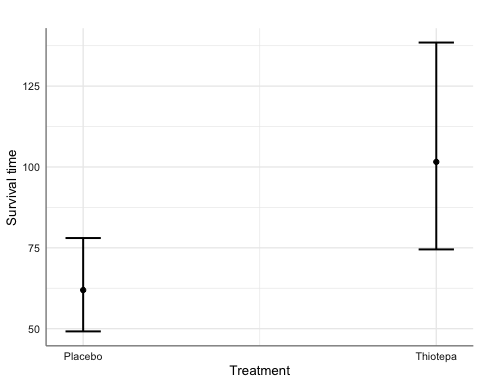## 5.2 Cox proportional hazards regression model_cox <- coxph(Surv(times, event) ~ rx, data = bladder) model_cox %>% glm_coef(labels = c("Treatment: Thiotepa/Placebo")) ParameterHazard ratioPr(>|z|) Treatment: Thiotepa/Placebo0.64 (0.44, 0.94) 0.02 Interpretation: Patients receiving Thiotepa are 64% less likely of dying than those in the Placebo group. model_cox %>% plot_model(type = "pred", terms = ~ rx, dot.size = 1.5, title = "") %>% gf_labs(x = "Treatment", title = "")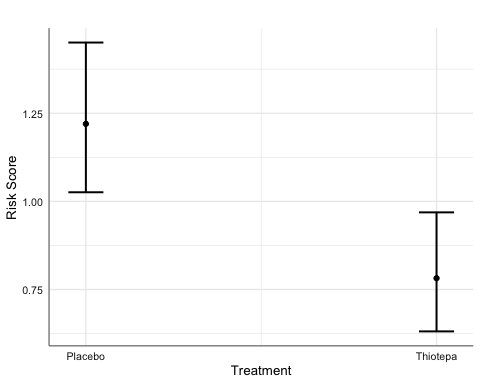# 6 Mixed Linear Regression Models ## 6.1 Continuous outcomes We look at the relationship between sex and age on the distance from the pituitary to the pterygomaxillary fissure (mm). library(nlme) Attaching package: 'nlme' The following objects are masked from 'package:ordinal': VarCorr, ranef The following object is masked from 'package:dplyr': collapse data(Orthodont) Orthodont <- Orthodont %>% var_labels( distance = "Pituitary distance (mm)", age = "Age (years)" ) We fit the model: model_lme <- lme(distance ~ Sex * I(age - mean(age, na.rm = TRUE)), random = ~ 1|Subject, method = "ML", data = Orthodont) model_lme %>% glm_coef(labels = c( "Constant", "Sex: female-male", "Age (years)", "Sex:Age interaction" ))  ParameterCoefficientPr(>|t|) Constant24.97 (24.03, 25.9)< 0.001 Sex: female-male-2.32 (-3.78, -0.86)0.005 Age (years)0.78 (0.63, 0.94)< 0.001 Sex:Age interaction-0.3 (-0.54, -0.07)0.015 Effect plot: model_lme %>% plot_model("pred", terms = age ~ Sex, show.data = TRUE, jitter = 0.1, dot.size = 1.5) %>% gf_labs(y = get_label(Orthodont$distance), x = "Age (years)", title = "")